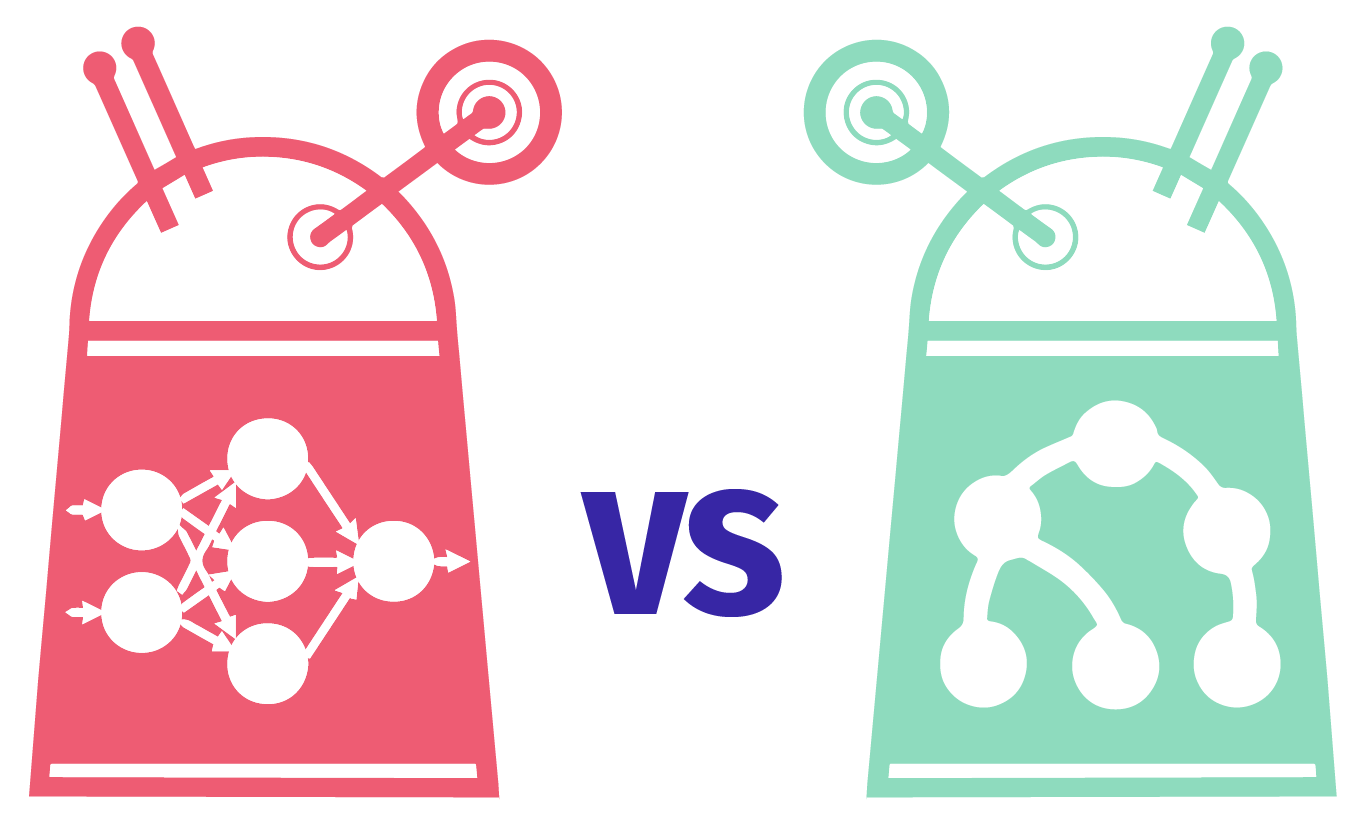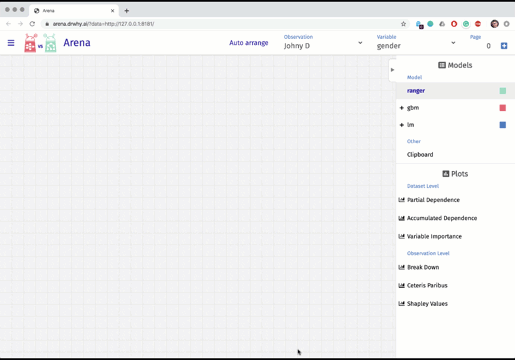## OverviewArena is an interactive tool that allows you to explore and compare any model regardless of its internal structure.

The arena can be run in two modes - live (R runs in the background and calculates all necessary explanations) and serverless (all necessary explanations are calculated earlier).

Using the Arena is trivially simple. An example with different levels of advancement is available at http://arenar.drwhy.ai/.## Installation

Install the ArenaR package from GitHub.

devtools::install_github("ModelOriented/ArenaR")

## How to use it

Examples generated with ArenaR

### Live version - fast for testing on huge data frames

library(arenar)
library(gbm)
library(DALEX)
library(dplyr)

# Create models and DALEX explainers
model_gbm100 <- gbm(m2.price ~ ., data = apartments, n.trees = 100)
expl_gbm100 <- explain(
model_gbm100,
data = apartments,
y = apartments$m2.price, label = "gbm [100 trees]" ) model_gbm500 <- gbm(m2.price ~ ., data = apartments, n.trees = 500) expl_gbm500 <- explain( model_gbm500, data = apartments, y = apartments$m2.price,
label = "gbm [500 trees]"
)

create_arena(live = TRUE) %>%
# Pushing explainers for each models
push_model(expl_gbm100) %>%
push_model(expl_gbm500) %>%
# Push dataframe of observations
push_observations(apartments) %>%
# Run server of default port and ip
run_server()


### Generating static files - easy to share

library(arenar)
library(gbm)
library(DALEX)
library(dplyr)

# Create models and DALEX explainers
model_gbm100 <- gbm(m2.price ~ ., data = apartments, n.trees = 100)
expl_gbm100 <- explain(
model_gbm100,
data = apartments,
y = apartments$m2.price, label = "gbm [100 trees]" ) model_gbm500 <- gbm(m2.price ~ ., data = apartments, n.trees = 500) expl_gbm500 <- explain( model_gbm500, data = apartments, y = apartments$m2.price,
label = "gbm [500 trees]"
)

# Take only few observations
observations <- apartments %>% filter(construction.year >= 2009)
# Observations' names are taken from rownames
rownames(observations) <- paste0(
observations$district, " ", observations$surface,
"m2 "
)

create_arena() %>%
# Pushing explainers for each models
push_model(expl_gbm100) %>%
push_model(expl_gbm500) %>%
# Push dataframe of observations
push_observations(observations) %>%
# Upload calculated arena files to Gist and open Arena in browser